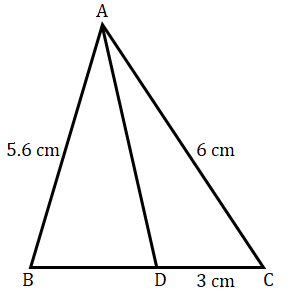"
">

# In a $Δ\ ABC$, $AD$ is the bisector of $∠\ A$, meeting side $BC$ at $D$. If $AB\ =\ 5.6\ cm$, $AC\ =\ 6\ cm$, and $DC\ =\ 3\ cm$, find $BC$."

Given:

In a $Δ\ ABC$, $AD$ is the bisector of $∠\ A$, meeting side $BC$ at $D$.

$AB\ =\ 5.6\ cm$, $AC\ =\ 6\ cm$, and $DC\ =\ 3\ cm$.

To do:

We have to find the measure of $BC$.

Solution:

$AD$ is the bisector of $∠\ A$, this implies,

$\angle BAD=\angle CAD$

We know that,

The internal bisector of an angle of a triangle divides the opposite side internally in the ratio of the corresponding sides containing the angle.

Therefore,

$\frac{AB}{AC} = \frac{BD}{DC}$

$\frac{5.6}{6} = \frac{BD}{3}$

$BD = \frac{3\times5.6}{6}$

$BD = \frac{5.6}{2}\ cm$

$BD=2.8\ cm$

From the figure,

$BC=BD+DC$

$BC=(2.8+3)\ cm=5.8\ cm$

The measure of $BC$ is $5.8\ cm$.

Updated on: 10-Oct-2022

27 Views